## Contents

A dask array looks and feels a lot like a numpy array. However, a dask array doesn’t directly hold any data. Instead, it symbolically represents the computations needed to generate the data. Nothing is actually computed until the actual numerical values are needed. This mode of operation is called “lazy”; it allows one to build up complex, large calculations symbolically before turning them over the scheduler for execution.

If we want to create a numpy array of all ones, we do it like this:

```import numpy as np
shape = (1000, 4000)
ones_np = np.ones(shape)
ones_np
```
```array([[1., 1., 1., ..., 1., 1., 1.],
[1., 1., 1., ..., 1., 1., 1.],
[1., 1., 1., ..., 1., 1., 1.],
...,
[1., 1., 1., ..., 1., 1., 1.],
[1., 1., 1., ..., 1., 1., 1.],
[1., 1., 1., ..., 1., 1., 1.]])
```

This array contains exactly 32 MB of data:

```ones_np.nbytes / 1e6
```
```32.0
```

Now let’s create the same array using dask’s array interface.

```import dask.array as da
ones = da.ones(shape)
ones
```
 Array Chunk 30.52 MiB 30.52 MiB (1000, 4000) (1000, 4000) 1 Tasks 1 Chunks float64 numpy.ndarray

The dask array representation reveals the concept of “chunks”. “Chunks” describes how the array is split into sub-arrays. We did not specify any chunks, so Dask just used one single chunk for the array. This is not much different from a numpy array at this point.

### Specifying Chunks¶

However, we could have split up the array into many chunks.source: Dask Array Documentation

There are several ways to specify chunks. In this lecture, we will use a block shape.

```chunk_shape = (1000, 1000)
ones = da.ones(shape, chunks=chunk_shape)
ones
```
 Array Chunk 30.52 MiB 7.63 MiB (1000, 4000) (1000, 1000) 4 Tasks 4 Chunks float64 numpy.ndarray

Notice that we just see a symbolic represetnation of the array, including its shape, dtype, and chunksize. No data has been generated yet. When we call `.compute()` on a dask array, the computation is trigger and the dask array becomes a numpy array.

```ones.compute()
```
```array([[1., 1., 1., ..., 1., 1., 1.],
[1., 1., 1., ..., 1., 1., 1.],
[1., 1., 1., ..., 1., 1., 1.],
...,
[1., 1., 1., ..., 1., 1., 1.],
[1., 1., 1., ..., 1., 1., 1.],
[1., 1., 1., ..., 1., 1., 1.]])
```

In order to understand what happened when we called `.compute()`, we can visualize the dask graph, the symbolic operations that make up the array

```ones.visualize()
```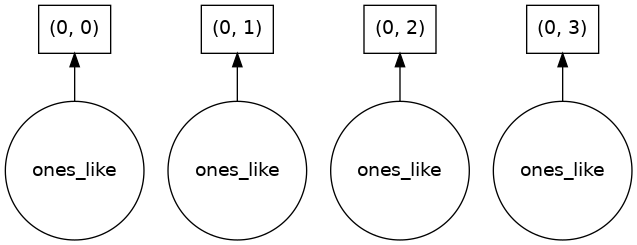Our array has four chunks. To generate it, dask calls `np.ones` four times and then concatenates this together into one array.

Rather than immediately loading a dask array (which puts all the data into RAM), it is more common to want to reduce the data somehow. For example

```sum_of_ones = ones.sum()
sum_of_ones.visualize()
```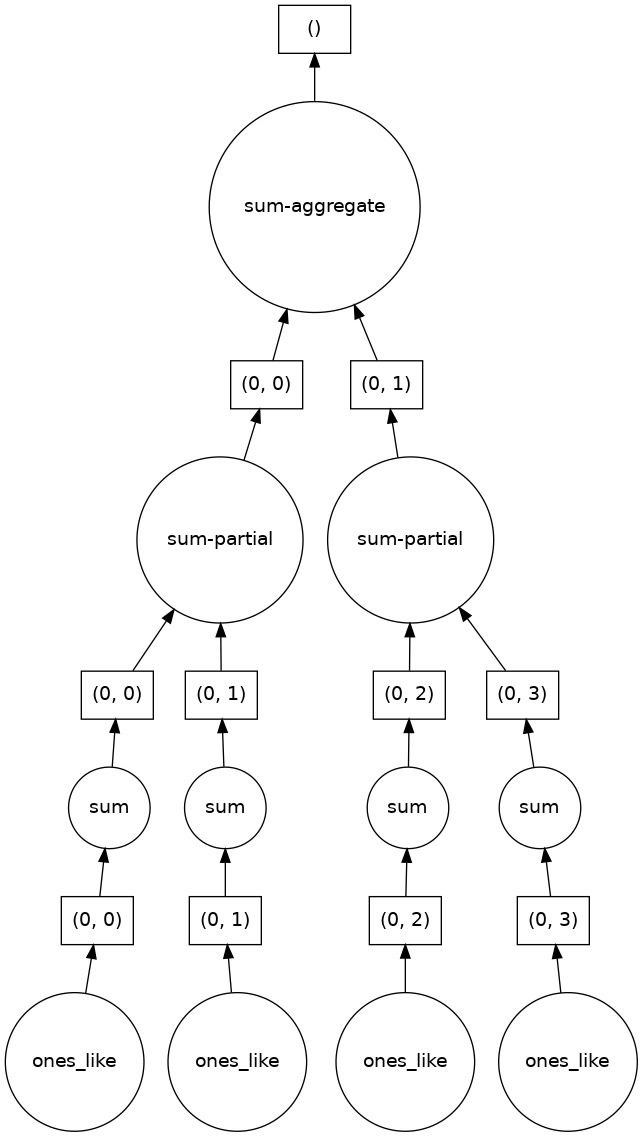Here we see dask’s strategy for finding the sum. This simple example illustrates the beauty of dask: it automatically designs an algorithm appropriate for custom operations with big data.

If we make our operation more complex, the graph gets more complex.

```fancy_calculation = (ones * ones[::-1, ::-1]).mean()
fancy_calculation.visualize()
```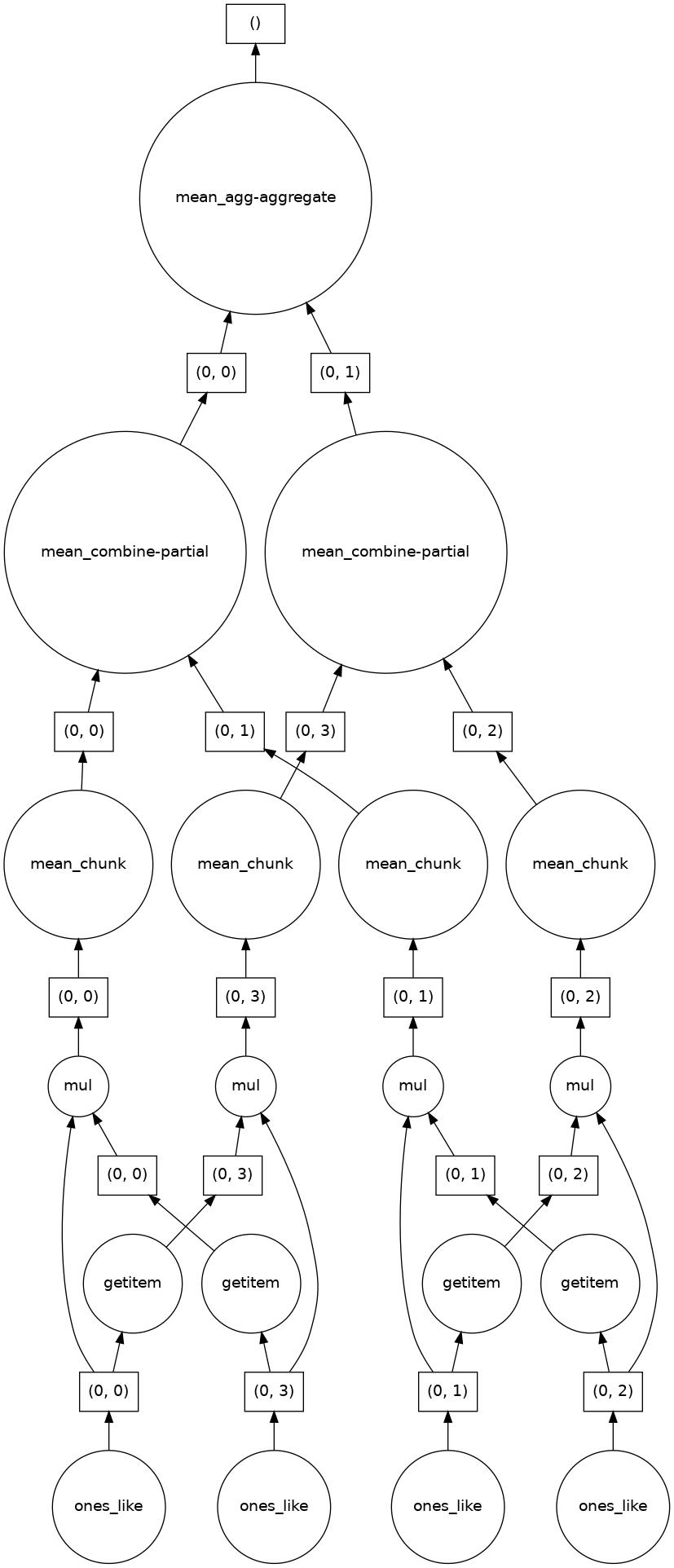### A Bigger Calculation¶

The examples above were toy examples; the data (32 MB) is nowhere nearly big enough to warrant the use of dask.

We can make it a lot bigger!

```bigshape = (200000, 4000)
big_ones = da.ones(bigshape, chunks=chunk_shape)
big_ones
```
 Array Chunk 5.96 GiB 7.63 MiB (200000, 4000) (1000, 1000) 800 Tasks 800 Chunks float64 numpy.ndarray
```big_ones.nbytes / 1e6
```
```6400.0
```

This dataset is 3.2 GB, rather MB! This is probably close to or greater than the amount of available RAM than you have in your computer. Nevertheless, dask has no problem working on it.

Do not try to `.visualize()` this array!

When doing a big calculation, dask also has some tools to help us understand what is happening under the hood

```from dask.diagnostics import ProgressBar

big_calc = (big_ones * big_ones[::-1, ::-1]).mean()

with ProgressBar():
result = big_calc.compute()
result
```
```[########################################] | 100% Completed |  2.4s
```
```1.0
```

### Reduction¶

All the usual numpy methods work on dask arrays. You can also apply numpy function directly to a dask array, and it will stay lazy.

```big_ones_reduce = (np.cos(big_ones)**2).mean(axis=0)
big_ones_reduce
```
 Array Chunk 31.25 kiB 7.81 kiB (4000,) (1000,) 3472 Tasks 4 Chunks float64 numpy.ndarray

Plotting also triggers computation, since we need the actual values

```from matplotlib import pyplot as plt
%matplotlib inline
plt.rcParams['figure.figsize'] = (12,8)
```
```plt.plot(big_ones_reduce)
```
```[<matplotlib.lines.Line2D at 0x7f18689fbb80>]
```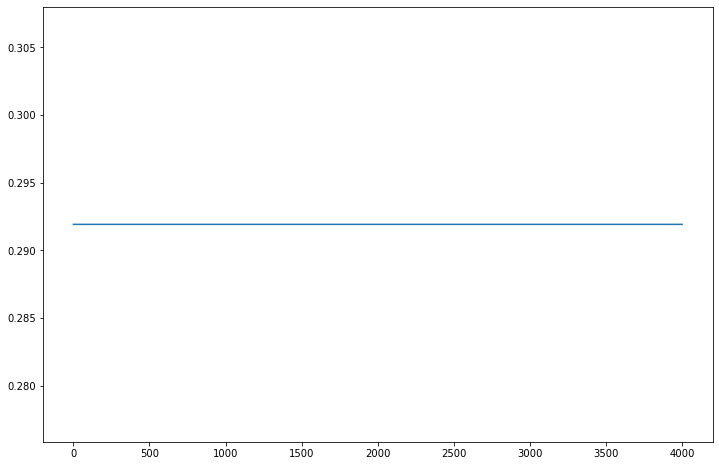## Distributed Clusters¶

Once we are ready to make a bigger calculation with dask, we can use a Dask Distributed cluster.

Warning

A common mistake is to move to distributed mode too soon. For smaller data, distributed will actually be much slower than the default multi-threaded scheduler or not using Dask at all. You should only use distributed when your data is much larger than what your computer can handle in memory.

### Local Cluster¶

A local cluster uses all the CPU cores of the machine it is running on. For our cloud-based Jupyterlab environments, that is just 2 cores–not very much. However, it’s good to know about.

```from dask.distributed import Client, LocalCluster
cluster = LocalCluster()
client = Client(cluster)
client
```

### Client

Client-0d54068d-5381-11ec-8481-f2f93f425864

 Connection method: Cluster object Cluster type: distributed.LocalCluster Dashboard: /user/rabernat/proxy/8787/status

### Cluster Info

Note that the “Dashboard” link will open a new page where you can monitor a computation’s progress.

```big_calc.compute()
```
```1.0
```

Here is another bigger calculation.

```random_values = da.random.normal(size=(2e8,), chunks=(1e6,))
hist, bins = da.histogram(random_values, bins=100, range=[-5, 5])
hist
```
 Array Chunk 800 B 800 B (100,) (100,) 668 Tasks 1 Chunks int64 numpy.ndarray
```# actually trigger the computation
hist_c = hist.compute()
```
```# plot results
x = 0.5 * (bins[1:] + bins[:-1])
width = np.diff(bins)
plt.bar(x, hist_c, width);
```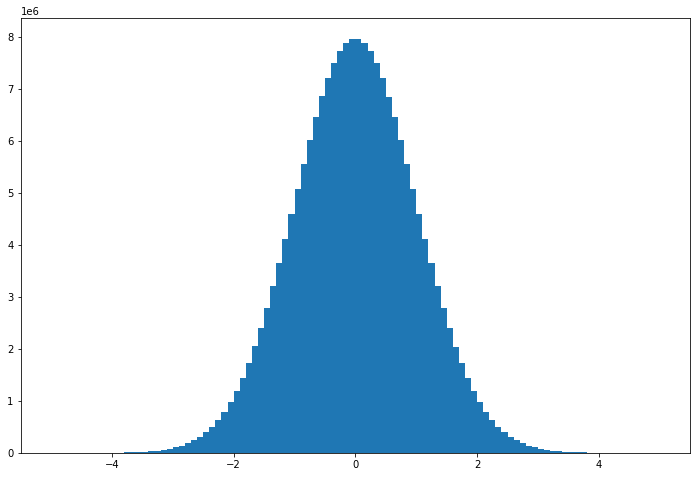### Dask Gateway - A Cluster in the Cloud¶

Pangeo Cloud makes it possible to launch a much larger cluster using many virtual machines in the cloud. This is beyond the scope of our class, but you can read more about it in the Pangeo Cloud docs

Xarray can automatically wrap its data in dask arrays. This capability turns xarray into an extremely powerful tool for Big Data earth science

To see this in action, we will open a dataset from the Pangeo Cloud Data Catalog - the Copernicus Sea Surface Height dataset.

```from intake import open_catalog
cat = open_catalog("https://raw.githubusercontent.com/pangeo-data/pangeo-datastore/master/intake-catalogs/ocean.yaml")
ds
```
```<xarray.Dataset>
Dimensions:    (time: 8901, latitude: 720, longitude: 1440, nv: 2)
Coordinates:
crs        int32 ...
lat_bnds   (time, latitude, nv) float32 dask.array<chunksize=(5, 720, 2), meta=np.ndarray>
* latitude   (latitude) float32 -89.88 -89.62 -89.38 ... 89.38 89.62 89.88
lon_bnds   (longitude, nv) float32 dask.array<chunksize=(1440, 2), meta=np.ndarray>
* longitude  (longitude) float32 0.125 0.375 0.625 0.875 ... 359.4 359.6 359.9
* nv         (nv) int32 0 1
* time       (time) datetime64[ns] 1993-01-01 1993-01-02 ... 2017-05-15
Data variables:
err        (time, latitude, longitude) float64 dask.array<chunksize=(5, 720, 1440), meta=np.ndarray>
sla        (time, latitude, longitude) float64 dask.array<chunksize=(5, 720, 1440), meta=np.ndarray>
ugos       (time, latitude, longitude) float64 dask.array<chunksize=(5, 720, 1440), meta=np.ndarray>
ugosa      (time, latitude, longitude) float64 dask.array<chunksize=(5, 720, 1440), meta=np.ndarray>
vgos       (time, latitude, longitude) float64 dask.array<chunksize=(5, 720, 1440), meta=np.ndarray>
vgosa      (time, latitude, longitude) float64 dask.array<chunksize=(5, 720, 1440), meta=np.ndarray>
Attributes: (12/43)
Conventions:                     CF-1.6
cdm_data_type:                   Grid
comment:                         Sea Surface Height measured by Altimetry...
contact:                         servicedesk.cmems@mercator-ocean.eu
creator_email:                   servicedesk.cmems@mercator-ocean.eu
...                              ...
summary:                         SSALTO/DUACS Delayed-Time Level-4 sea su...
time_coverage_duration:          P1D
time_coverage_end:               1993-01-01T12:00:00Z
time_coverage_resolution:        P1D
time_coverage_start:             1992-12-31T12:00:00Z
title:                           DT merged all satellites Global Ocean Gr...```

Note that the values are not displayed, since that would trigger computation.

```ds.sla.plot()
```
```<matplotlib.collections.QuadMesh at 0x7f17ae3e4f10>
```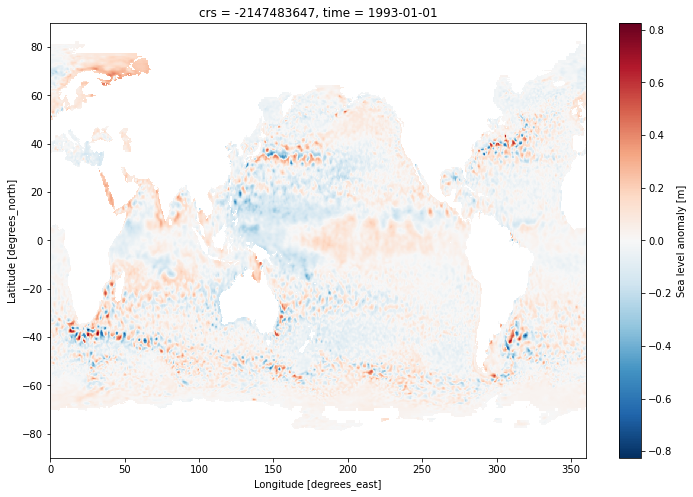```# the computationally intensive step
```sla_timeseries.plot(label='full data')